Test: Forecasting Routing & Scheduling - 1

# Test: Forecasting Routing & Scheduling - 1

Test Description

## 20 Questions MCQ Test RRB JE for Mechanical Engineering | Test: Forecasting Routing & Scheduling - 1

Test: Forecasting Routing & Scheduling - 1 for Mechanical Engineering 2022 is part of RRB JE for Mechanical Engineering preparation. The Test: Forecasting Routing & Scheduling - 1 questions and answers have been prepared according to the Mechanical Engineering exam syllabus.The Test: Forecasting Routing & Scheduling - 1 MCQs are made for Mechanical Engineering 2022 Exam. Find important definitions, questions, notes, meanings, examples, exercises, MCQs and online tests for Test: Forecasting Routing & Scheduling - 1 below.
Solutions of Test: Forecasting Routing & Scheduling - 1 questions in English are available as part of our RRB JE for Mechanical Engineering for Mechanical Engineering & Test: Forecasting Routing & Scheduling - 1 solutions in Hindi for RRB JE for Mechanical Engineering course. Download more important topics, notes, lectures and mock test series for Mechanical Engineering Exam by signing up for free. Attempt Test: Forecasting Routing & Scheduling - 1 | 20 questions in 60 minutes | Mock test for Mechanical Engineering preparation | Free important questions MCQ to study RRB JE for Mechanical Engineering for Mechanical Engineering Exam | Download free PDF with solutions
 1 Crore+ students have signed up on EduRev. Have you?
Test: Forecasting Routing & Scheduling - 1 - Question 1

### Which one of the following forecasting techniques is not suited for making forecasts for planning production schedules in the short range?

Detailed Solution for Test: Forecasting Routing & Scheduling - 1 - Question 1

Moving, average, Exponential moving average is used for short range.
Regression is used for short and medium range.  Delphi is used for long range forecasting.

*Multiple options can be correct
Test: Forecasting Routing & Scheduling - 1 - Question 2

### Production flow analysis (PFA) is a method of identifying part families that uses data from

Test: Forecasting Routing & Scheduling - 1 - Question 3

### A moving average system is used for forecasting weekly demand. F1(t) and F2(t) are sequences of forecasts with parameters m1 and m2, respectively, where m1 and m2 (m1 > m2) denote the numbers of weeks over which the moving averages are taken. The actual demand shows a step increase from d1 to d2 at a certain time. Subsequently,

Detailed Solution for Test: Forecasting Routing & Scheduling - 1 - Question 3

Since, m1>m2 the weightage of the latest demand would be more in F2 (t). So, F2 (t) is sensitive data.

Hence F2 (t) will attain the value d2 before F1 (t).

Test: Forecasting Routing & Scheduling - 1 - Question 4

A manufacturing shop processes sheet metal jobs, wherein each job must pass through two machines (M1 and M2, in that order). The processing time (in hours) for these jobs is: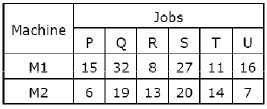The optimal make-span (in hours) of the shop is:

Detailed Solution for Test: Forecasting Routing & Scheduling - 1 - Question 4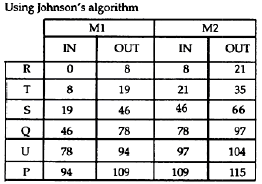Test: Forecasting Routing & Scheduling - 1 - Question 5

When using a simple moving average to forecast demand, one would

Test: Forecasting Routing & Scheduling - 1 - Question 6

Four jobs are to be processed on a machine as per data listed in the table.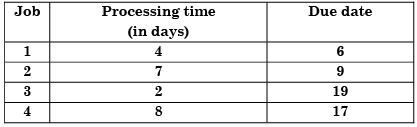If the Earliest Due-date (EDD) rule is used to sequence the jobs, the number of jobs delayed is:

Detailed Solution for Test: Forecasting Routing & Scheduling - 1 - Question 6

Alternating According to EDD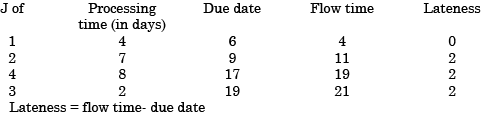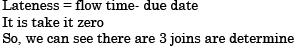Test: Forecasting Routing & Scheduling - 1 - Question 7

Four jobs are to be processed on a machine as per data listed in the table.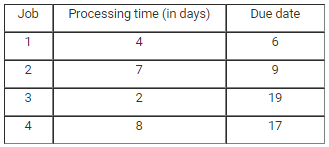Using the Shortest Processing Time (SPT) rule, total tardiness is

Detailed Solution for Test: Forecasting Routing & Scheduling - 1 - Question 7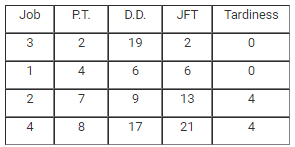Since, negative tardiness is not considered as delay i.e. considered as 0 tardiness.

So, Total tardiness =  0 + 0 + 4 + 4 = 8

Test: Forecasting Routing & Scheduling - 1 - Question 8

Which of the following forecasting methods takes a fraction of forecast error into account for the next period forecast?

Test: Forecasting Routing & Scheduling - 1 - Question 9

Routing in production planning and control refers to the

Test: Forecasting Routing & Scheduling - 1 - Question 10

The demand and forecast for February are 12000 and 10275, respectively. Using single exponential smoothening method (smoothening coefficient = 0.25), forecast for the month of March is:

Detailed Solution for Test: Forecasting Routing & Scheduling - 1 - Question 10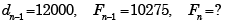According to single exponential smoothing method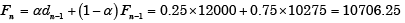Test: Forecasting Routing & Scheduling - 1 - Question 11

The routing function in a production system design is concerned with.

Test: Forecasting Routing & Scheduling - 1 - Question 12

The sales of a product during the last four years were 860, 880, 870 and 890 units. The forecast for the fourth year was 876 units. If the forecast for the fifth year, using simple exponential smoothing, is equal to the forecast using a three period moving average, the value of the exponential smoothing constant a is:

Detailed Solution for Test: Forecasting Routing & Scheduling - 1 - Question 12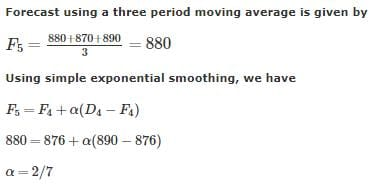.

Test: Forecasting Routing & Scheduling - 1 - Question 13

Consider the following statements:

Scheduling

1. Is a general timetable of manufacturing
4. Machines according to their capacity

Which of the statements given above are correct?

Test: Forecasting Routing & Scheduling - 1 - Question 14

For a product, the forecast and the actual sales for December 2002 were 25 and 20 respectively. If the exponential smoothing constant (α) is taken as 0.2, then forecast sales for January, 2003 would be:

Detailed Solution for Test: Forecasting Routing & Scheduling - 1 - Question 14

Use new forecast = old forecast + α (actual demand – old forecast)

Test: Forecasting Routing & Scheduling - 1 - Question 15

Consider the following statements:

1. Preparation of master production schedule is an iterative process
2. Schedule charts are made with respect to jobs while load charts are made with respect to machines
3. MRP is done before master production scheduling

Which of the statements given above are correct?

Test: Forecasting Routing & Scheduling - 1 - Question 16

The sales of cycles in a shop in four consecutive months are given as 70, 68, 82, and 95. Exponentially smoothing average method with a smoothing factor of 0.4 is used in forecasting. The expected number of sales in the next month is:

Detailed Solution for Test: Forecasting Routing & Scheduling - 1 - Question 16

Let expected number of sales in the next month = ut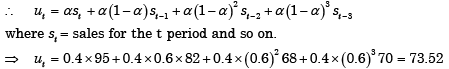Test: Forecasting Routing & Scheduling - 1 - Question 17

Which of the following factors are to be considered for production scheduling?

1. Sales forecast
2. Component design
3. Route sheet
4. Time stand ards

Select the correct answer using the codes given below:

Test: Forecasting Routing & Scheduling - 1 - Question 18

In a forecasting model, at the end of period 13, the forecasted value for period 14 is 75. Actual value in the periods 14 to 16 are constant at 100. If the assumed simple exponential smoothing parameter is 0.5, then the MSE at the end of period 16 is:

Detailed Solution for Test: Forecasting Routing & Scheduling - 1 - Question 18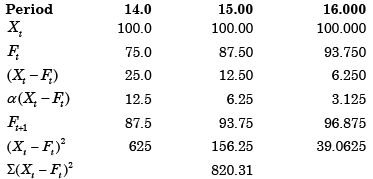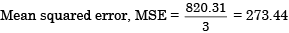Test: Forecasting Routing & Scheduling - 1 - Question 19

Assertion (A): Planning and scheduling of job order manufacturing differ from planning and scheduling of mass production manufacturing.

Reason (R): In mass production manufacturing, a large variety of products are manufactured in large quantity.

Test: Forecasting Routing & Scheduling - 1 - Question 20

The most commonly used criteria for measuring forecast error is:

## RRB JE for Mechanical Engineering

5 videos|9 docs|148 tests
 Use Code STAYHOME200 and get INR 200 additional OFF Use Coupon Code
Information about Test: Forecasting Routing & Scheduling - 1 Page
In this test you can find the Exam questions for Test: Forecasting Routing & Scheduling - 1 solved & explained in the simplest way possible. Besides giving Questions and answers for Test: Forecasting Routing & Scheduling - 1, EduRev gives you an ample number of Online tests for practice

## RRB JE for Mechanical Engineering

5 videos|9 docs|148 tests